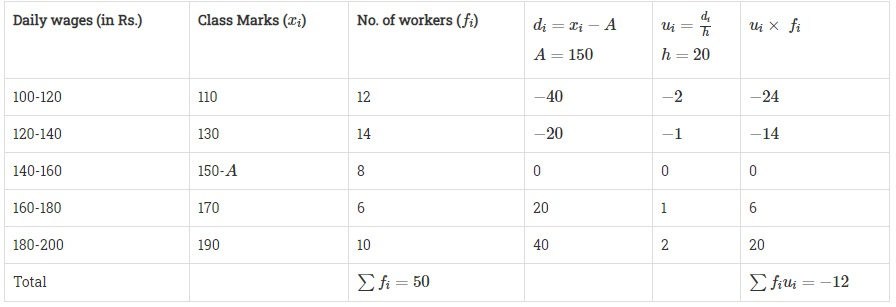# Consider the following distribution of daily wages of 50 workers of a factory.Daily wages (in Rs).100-120120-140140-160160-180180-200Number of workers:12148610Find the mean daily wages of the workers of the factory by using an appropriate method."

Given:

Daily wages of 50 workers of a factory.

To do:

We have to find the mean daily wages of the workers of the factory.

Solution:

Let the assumed mean be $A=150$We know that, Mean $=A+h \times \frac{\sum{f_iu_i}}{\sum{f_i}}$

Therefore,

Mean $=150+20\times\frac{-12}{50}$

$=150-\frac{24}{5}$

$=150-4.8$

$=145.2$

The mean daily wages of the workers of the factory is $Rs.\ 145.20$.